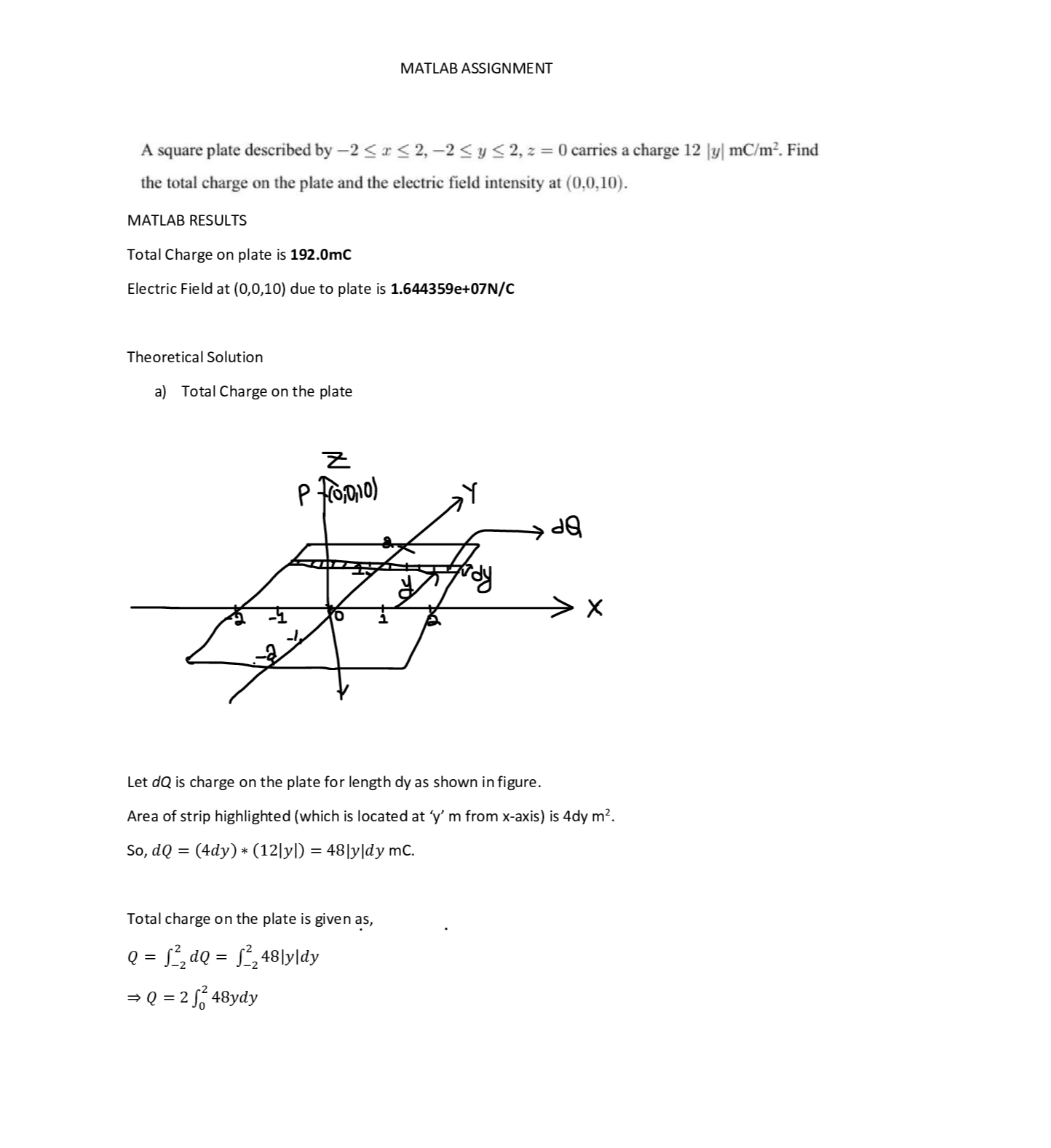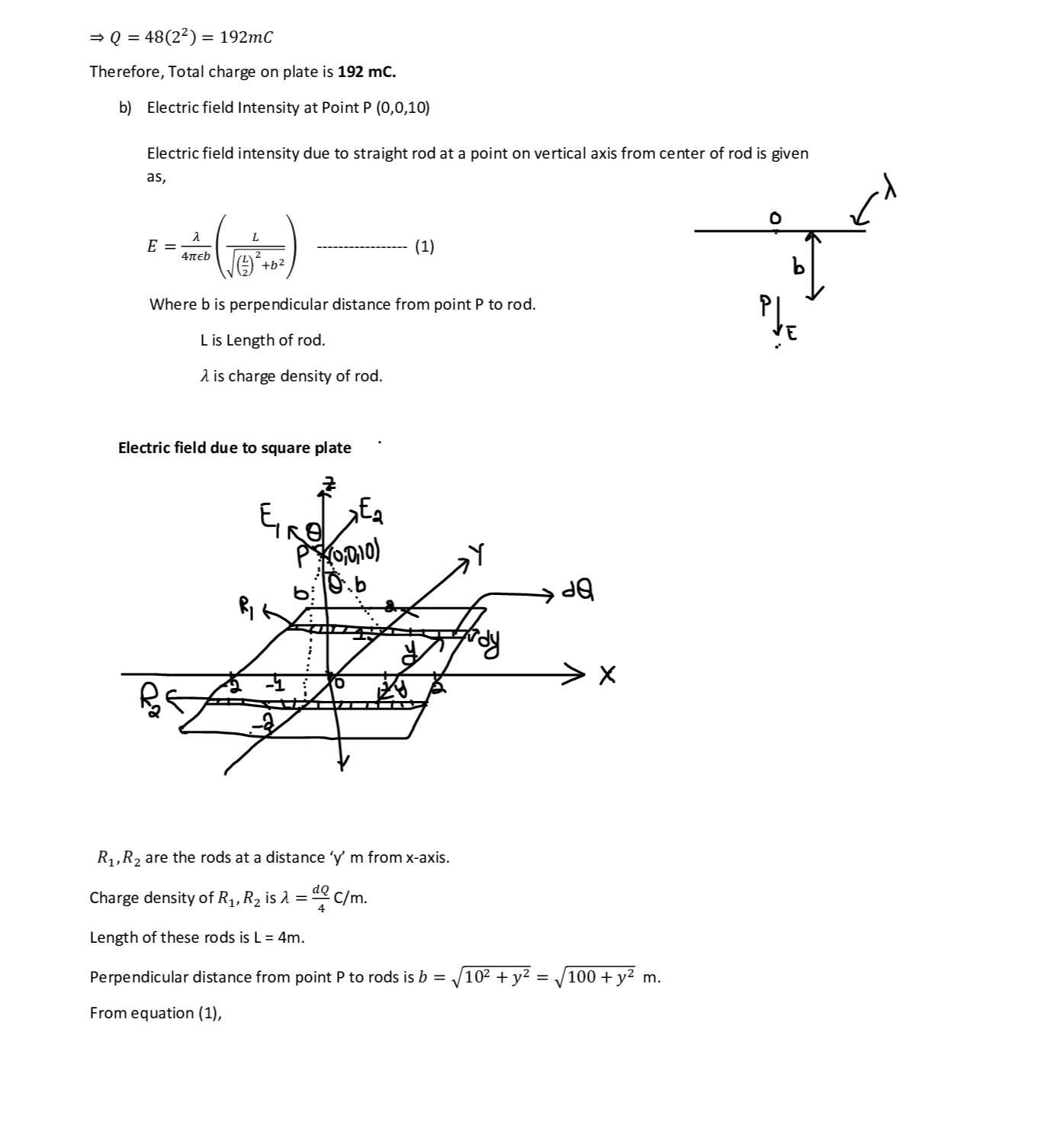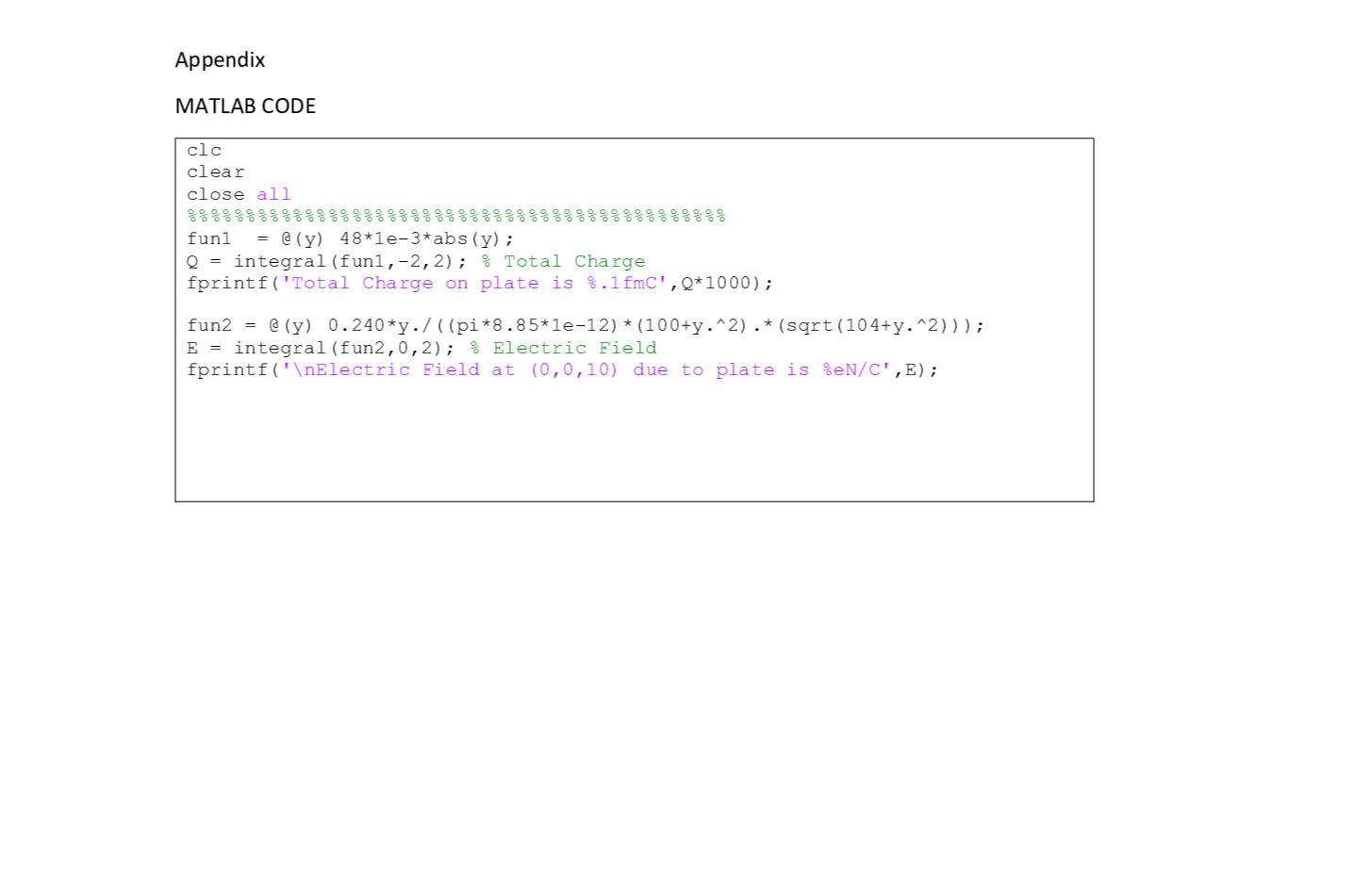Question

Matlab

A square plate described by -2 < r< 2, -2 <y < 2, z = 0 carries a charge 12 |y| mC/m?. Find the total charge on the plate and the electric field intensity at (0,0,10).

Use MATLAB to compute the answers of the problem. Print the answers on the command window.And support your results by verifying the answers theoretically. Discuss the results.

MATLAB hint: There is more than one way to compute the integration in MATLAB. You can compute the integration numerically using the 'integral' function. Also you can compute the integration symbolically using the int' function and convert it to numerical value, or by doing it directly using the 'ypa integral' function. And you can do it the hard way using 'for loops'.

In Moodle, submit a single PDF file that includes the MATLAB results, theoretical solution, and discussion. Also include a formatted copy of your code (i.e., do not take screenshots of the code,copy the text) at the end of the document as an appendix. Also submit your code as an m-file.Verified### Question 44520Matlab

10.An infinite length line carries current I in the +directional on the z-axis, and this is surrounded by an infinite length cylindrical shell (centered about the z-axis)of radius a carrying the return current I in the -azdirection as a surface current. Find expressions for the magnetic field intensity everywhere. If the current is 1.0A and the radius a is 2.0 cm, plot the magnitude of H versus radial distance from the z-axis from 0.1 cm to 4 cm.

### Question 44519Matlab

9. An infinitesimally thin metallic cylindrical shell of radius4.0 cm is centered on the z-axis and carries an evenly distributed current of 10.0 mA in the +az direction. (a)Determine the value of the surface current density on the
conductive shell and (b) plot H as a function of radial distance from the z-axis over the range 0 < 22< 12 cm.

### Question 44518Matlab

8. Given a 3.0 mm radius solid wire centered on the z-axis with an evenly distributed 2.0 amps of current in the +azdirection, plot the magnetic field intensity H versus radial distance from the z-axis over the range 0 <O< 9 mm.

### Question 44517Matlab

7. How close do you have to be to the middle of a finite length of current-carrying line before it appears infinite in length? Consider Hf(0, a, 0) is the field for the finite line of length 2h centered on the z-axis, and that Hi(0, a, 0) is the field for an infinite length line of current on the z-axis.In both cases consider current I in the +az direction. Plot Hf/Hi vs h/a.

### Question 44516Matlab

6. A thick-walled spherical shell, with inner radius 2.00 cm and outer radius 4.00 cm, has an evenly distributed 12.0nC charge. Plot Dr as a function of radial distance from the origin over the range 0 <r< 10 cm.

### Question 44515Matlab

1. MATLAB: Suppose you have a segment of line charge of length 2L centered on the z-axis and having a charge distribution BL. Compare the electric field intensity at a point on the y-axis a distance d from the origin with the electric field at that point assuming the line charge is of infinite length. The ratio of E for the segment to E for the infinite line is to be plotted versus the ratio L/d usingMATLAB.

### Question 44514Matlab

4. Given a 3.00 mm radius solid wire centered on the z-axis with an evenly distributed 2.00 coulombs of charge perimeter length of wire, plot the electric flux density DB versus radial distance from the z-axis over the range 0<<9 mm.

### Question 44513Matlab

3. : MATLAB: Consider a sphere with charge density Bv =120 nC/m3 centered at the origin with a radius of 2.00 m.Now, remove the top half of the sphere, leaving a hemisphere below the x-y plane. Find the electric field intensity at the point P(8.00m,0.00,0.00). (Hint: seeMATLAB 2.4, and consider that your answer will now have two field components.)

### Question 44512Matlab

2. MATLAB: Consider a rectangular volume with 0.00 < xs4.00 m, 0.00 < y< 5.00 m and -6.00 m < z< 0.00 with charge density Bv = 40.0 nC/m3. Find the electric field intensity at the point P(0 00 .0 00 20.0m)

### Question 44511Matlab

1. MATLAB: Suppose you have a segment of line charge of length 2L centered on the z-axis and having a charge distribution BL. Compare the electric field intensity at a point on the y-axis a distance d from the origin with the electric field at that point assuming the line charge is of infinite length. The ratio of E for the segment to E for the infinite line is to be plotted versus the ratio L/d usingMATLAB.

### Submit query